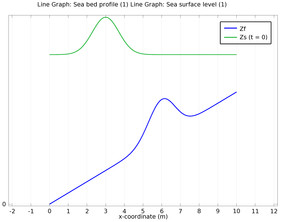# アプリケーションギャラリ

## The Shallow Water Equations

Application ID: 202

The Shallow Water equations are frequently used for modeling both oceanographic and atmospheric fluid flow. Models of such systems lead to the prediction of areas eventually affected by pollution, coast erosion and polar ice-cap melting.

Comprehensive modeling of such phenomena using physical descriptions such as the Navier-Stokes equations can often be problematic, due to the scale of the modeling domains as well as resolving free surfaces. The Shallow Water equations, of which there are a number of representations, provide an easier description of such phenomena. This 1D model investigates the settling of a wave over a variable bed as a function of time.This model example illustrates applications of this type that would nominally be built using the following products: